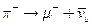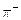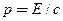Chapter 33, Problem 47PE

Chapter
Section
Textbook Problem

Integrated ConceptsThe primary decay mode for the negative pion is(a) What is the energy release in MeV in this decay? (b) Using conservation of momentum, how much energy does each of the decay products receive, given theis at rest when it decays? You may assume the muon antineutrino is massless and has momentumjust like a photon.

To determine

(a)

Amount of energy released in the decay of negative pion given by, πμ+ν¯μ

Explanation

Given:

Given reaction is πμ+ν¯μ

Formula used:

Energy released in a decay process

= Rest energy of initial particle − sum of energies of decay products

Calculation:

In this problem the negative pion decays by πμ+ν¯μ

Rest energy of π particle =139

To determine

(b)

The amount of energy received by decay products.

Still sussing out bartleby?

Check out a sample textbook solution.

See a sample solution

The Solution to Your Study Problems

Bartleby provides explanations to thousands of textbook problems written by our experts, many with advanced degrees!

Get Started

Vitamin K is necessary for the synthesis of key bone proteins. T F

Nutrition: Concepts and Controversies - Standalone book (MindTap Course List)

What evidence can you give that granulation is caused by convection?

Horizons: Exploring the Universe (MindTap Course List)

25-20 What constitutes the backbone of DNA?

Introduction to General, Organic and Biochemistry

9.36 Define the term calibration.

Chemistry for Engineering Students